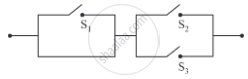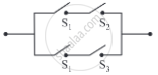# Check whether the following switching circuits are logically equivalent - Justify. (i) (ii) - Mathematics and Statistics

Sum

Check whether the following switching circuits are logically equivalent - Justify.

(i)(ii)#### Solution

Let p: the switch S1 is closed
q: the switch S2 is closed
r: the switch S3 is closed
The symbolic form of the given switching circuits is p ∧ (q ∨ r) and (p ∧ q) ∨ (p ∧ r) respectively.
By Distributive Law, p ∧ (q ∨ r) ≡ (p ∧ q) ∨ (p ∧ r)
Hence, the given switching circuits are logically equivalent.

Concept: Application of Logic to Switching Circuits
Is there an error in this question or solution?

#### APPEARS IN

Balbharati Mathematics and Statistics 1 (Arts and Science) 12th Standard HSC Maharashtra State Board
Chapter 1 Mathematical Logic
Miscellaneous Exercise 1 | Q 14.1 | Page 35